# Overview: the JavaScript Number toFixed Methodself.__wrap_n=self.__wrap_n||(self.CSS&&CSS.supports("text-wrap","balance")?1:2);self.__wrap_b=(e,r,t)=>{t=t||document.querySelector(`[data-br="\${e}"]`);let n=t.parentElement,a=s=>t.style.maxWidth=s+"px";t.style.maxWidth="";let o,i=n.clientWidth,l=n.clientHeight,c=i/2-.25,f=i+.5;if(i){for(a(c),c=Math.max(t.scrollWidth,c);c+1<f;)o=Math.round((c+f)/2),a(o),n.clientHeight===l?f=o:c=o;a(f*r+i*(1-r))}t.__wrap_o||typeof ResizeObserver<"u"&&(t.__wrap_o=new ResizeObserver(()=>{self.__wrap_b(0,+t.dataset.brr,t)})).observe(n)};self.__wrap_n!=1&&self.__wrap_b(":Rj0pm:",1)

November 6, 2023

The `toFixed` method in JavaScript formats a number using fixed-point notation, letting you specify the number of digits after the decimal point. It’s great for displaying currency or other numbers that require a certain precision.

## What is the toFixed method in JavaScript?

The `toFixed` method is a part of the Number prototype in JavaScript. It performs a type conversion if necessary, forcing a number to conform to a specified format where the number of digits after the decimal point is fixed. The method returns a string representation of a number that does not round the number until it converts it to the final string form.

## How to use toFixed in JavaScript

### Syntax

`num.toFixed([digits])`
• `num` is the original number you want to format.
• `digits` (optional) is an integer specifying the number of digits to appear after the decimal point; this can be a value between 0 and 20, inclusive, although implementations may optionally support a larger range of values.

### Basic example

```const number = 123.456;
console.log(number.toFixed(2)); // "123.46"```

## Handling 'toFixed is not a function' error

### Why this error occurs

The "toFixed is not a function" error occurs when the `toFixed` method is called on something that is not a number. Since `toFixed` is a method of Number objects, calling it on a non-number type like a string or an object will result in this error.

### How to fix it

Ensure that the variable on which you're calling `toFixed` is indeed a number.

```let value = "123.456";
value = Number(value).toFixed(2); // "123.46" if the conversion is successful```

However, if the string or object cannot be converted to a number, `Number()` will return `NaN`, and `toFixed` will still fail. Always validate your data before attempting to use `toFixed`.

## Dealing with precision and rounding

`toFixed` will round the number to the nearest value, depending on the number of digits specified.

```const number = 123.456789;
console.log(number.toFixed(3)); // "123.457"```

If the number is exactly halfway between two possible rounded values, the method rounds towards the nearest even number (this is known as "bankers' rounding").

## Rounding with toFixed

The method's rounding can sometimes be counterintuitive due to the floating-point arithmetic used in JavaScript.

```const number = 2.005;
console.log(number.toFixed(2)); // "2.00" instead of "2.01"```

This is a known issue due to the IEEE 754 standard used by JavaScript for number representation, which can lead to such rounding errors. One approach to address this is by adjusting the number before applying `toFixed`.

```const number = 2.005;
console.log((number + Number.EPSILON).toFixed(2)); // "2.01"```

## Limitations and considerations

• Precision: While `toFixed` is useful, it's not suitable for all scenarios, especially where scientific calculations with variable precision are involved.
• Rounding errors: The rounding algorithm can introduce small errors, which are especially problematic in financial calculations.
• Performance: For high-performance applications, the overhead of converting numbers to strings should be considered.

## What does toFixed do in JavaScript

In essence, `toFixed` ensures numerical output is formatted to a fixed number of decimal places, converted to a string, making it indispensable for formatting prices and other fixed-decimal values.

## Using toFixed with different data types

### Numbers

```const num = 10;
console.log(num.toFixed(2)); // "10.00"```

### Strings that can convert to numbers

```const numStr = "15.6789";
console.log(parseFloat(numStr).toFixed(1)); // "15.7"```

### Objects and non-numeric values

Objects or non-numeric values require conversion before `toFixed` can be used.

```const obj = { value: 67.89 };
// This will throw an error
console.log(obj.value.toFixed(1)); // Uncaught TypeError: obj.value.toFixed is not a function
// Correct approach
console.log(Number(obj.value).toFixed(1)); // "67.9"```

## Formatting numbers for localization

For localized number formatting, the `Intl.NumberFormat` constructor provides language-sensitive number formatting.

```const number = 1234.56;
console.log(new Intl.NumberFormat('en-US').format(number)); // "1,234.56"```

## Potential for unexpected results and error handling

Using `toFixed` without proper error handling can lead to unexpected results, especially if you're dealing with non-numeric values or complex operations.

```const num = null;
if (num !== null && num !== undefined) {
console.log(num.toFixed(2));
} else {
console.log('Number is undefined or null.');
}```

It's essential to verify the type and value before calling `toFixed` to prevent runtime errors.

# Ship faster, worry less with Basedash

You're busy enough with product work to be weighed down building, maintaining, scoping and developing internal apps and admin panels. Forget all of that, and give your team the admin panel that you don't have to build. Launch in less time than it takes to run a standup.

You're busy enough with product work to be weighed down building, maintaining, scoping and developing internal apps and admin panels. Forget all of that, and give your team the admin panel that you don't have to build. Launch in less time than it takes to run a standup.

You're busy enough with product work to be weighed down building, maintaining, scoping and developing internal apps and admin panels. Forget all of that, and give your team the admin panel that you don't have to build. Launch in less time than it takes to run a standup.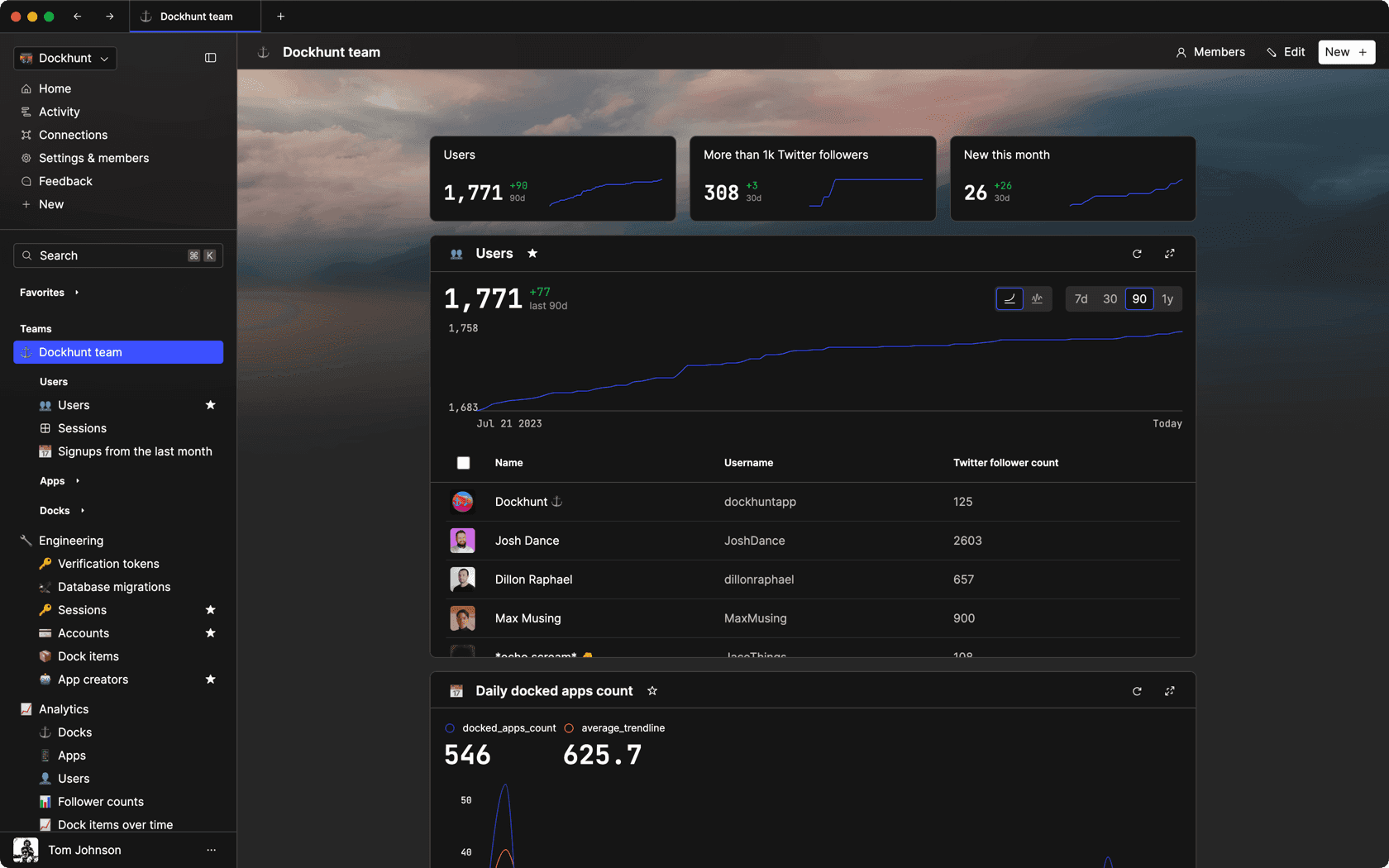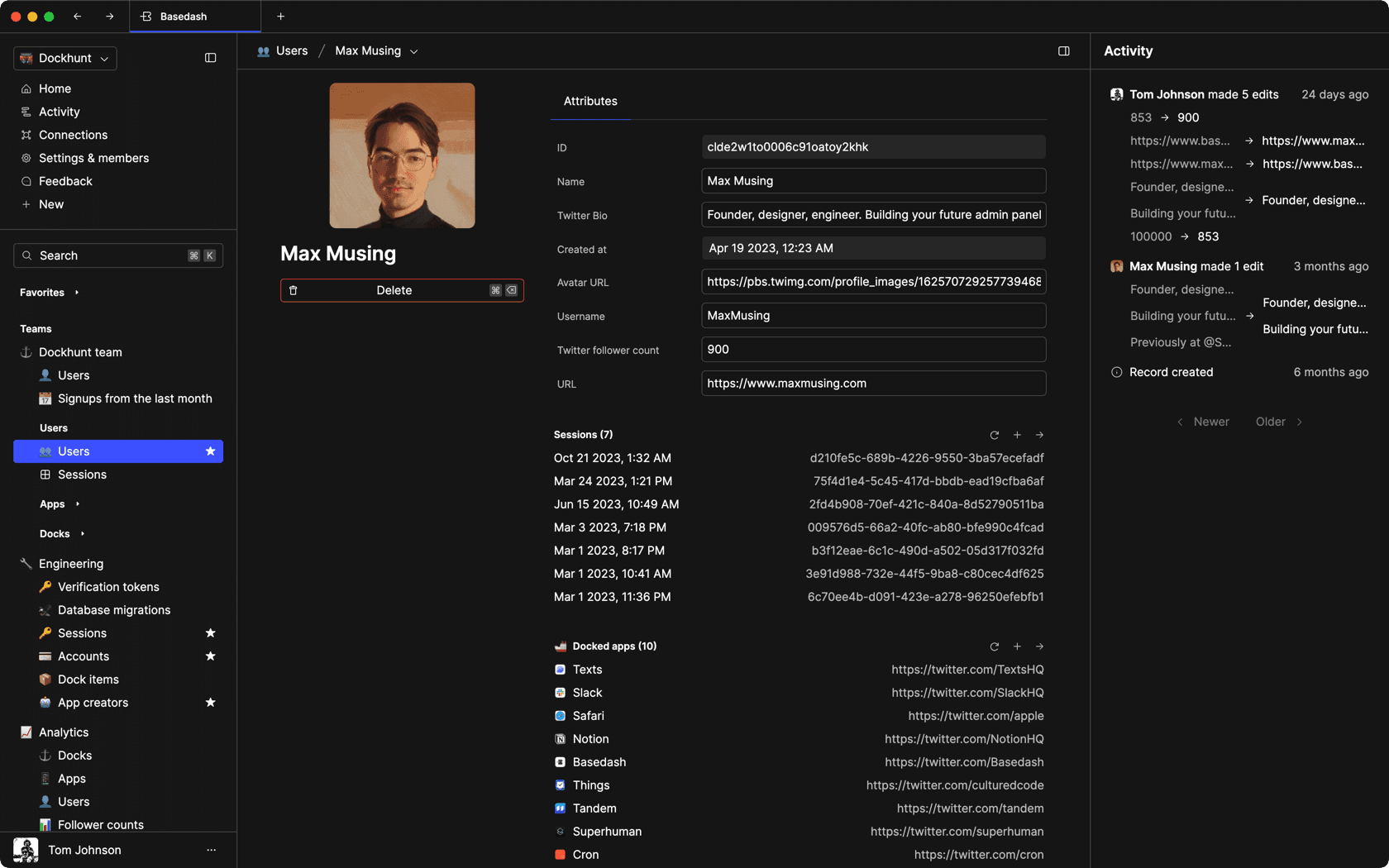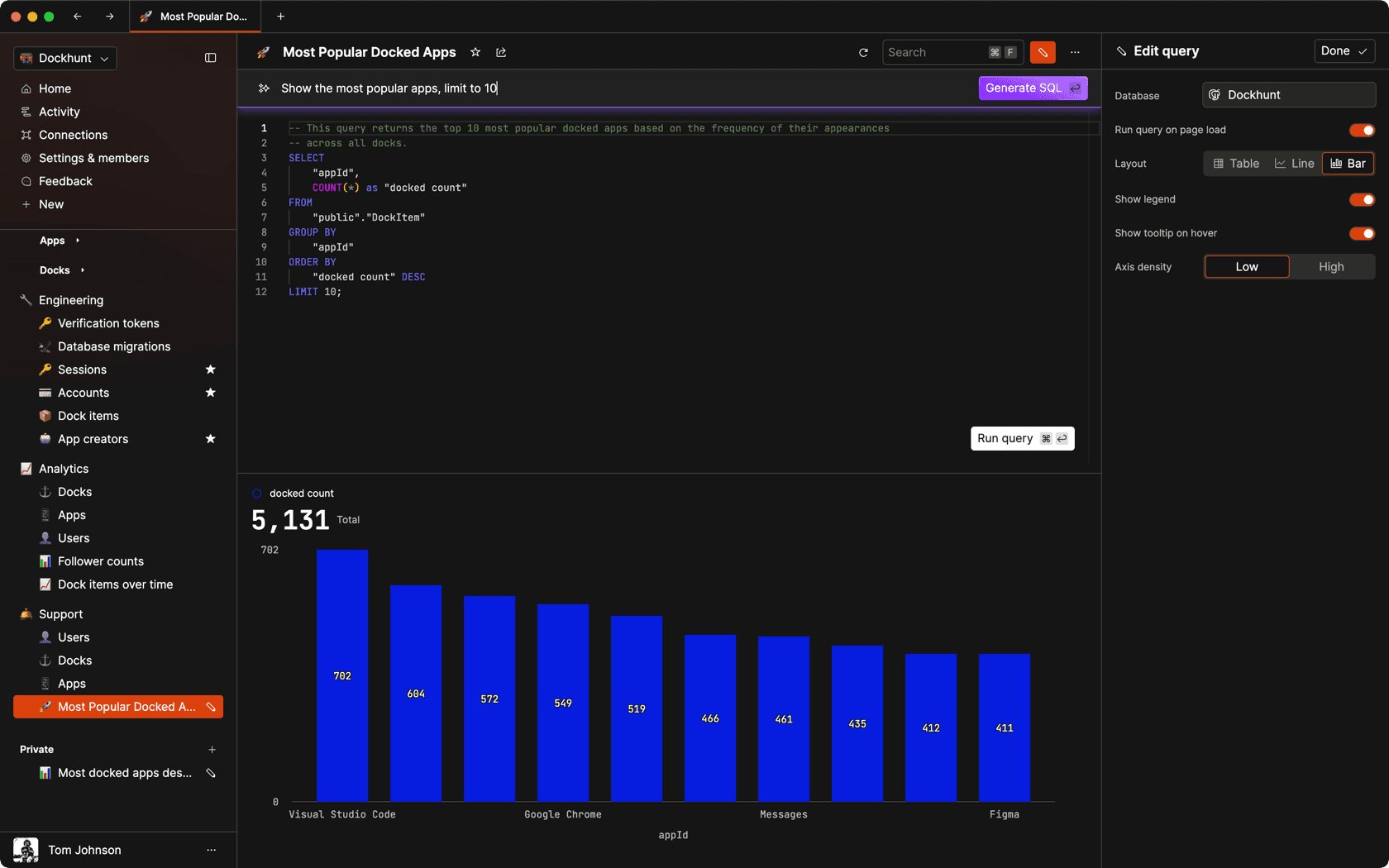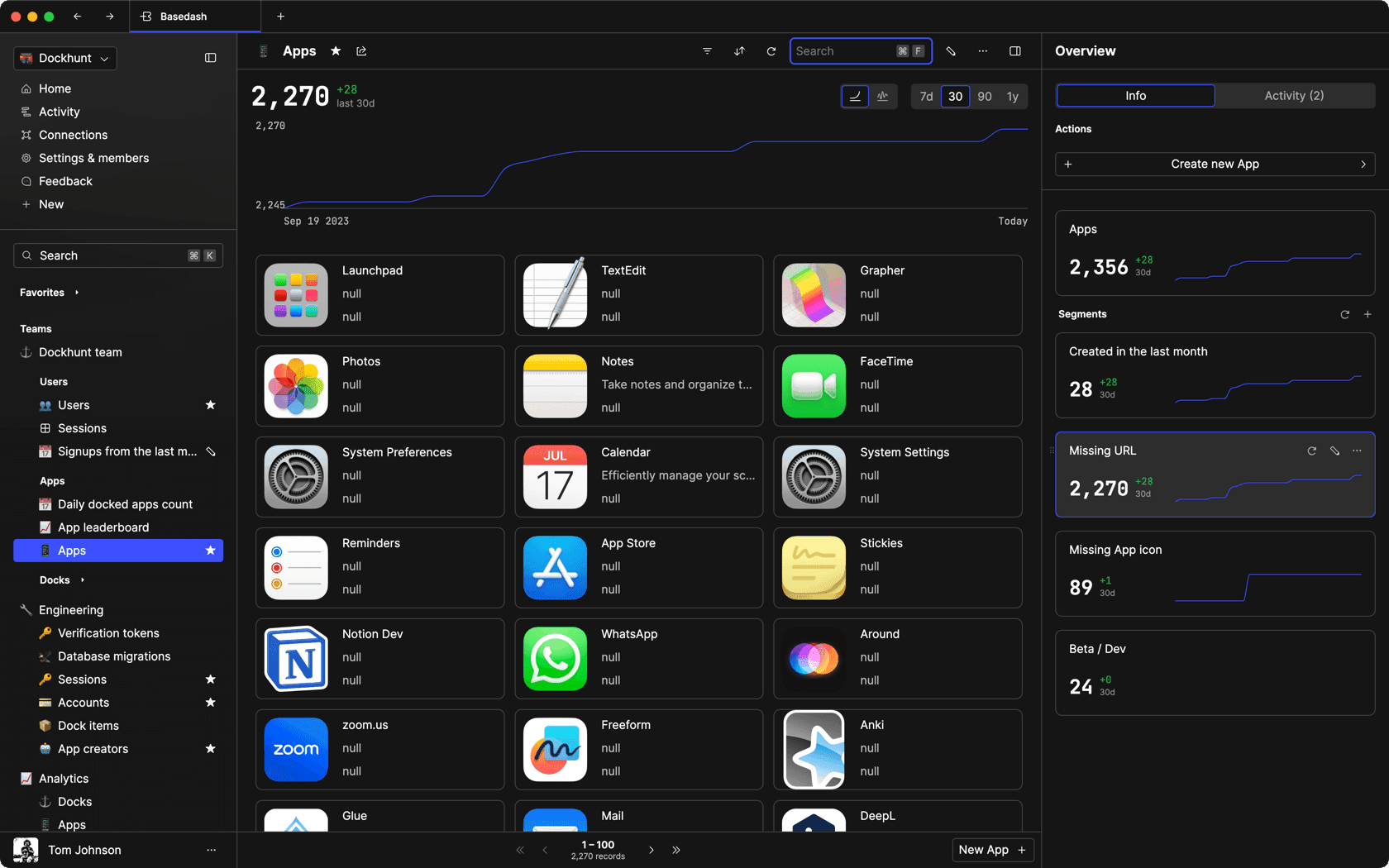### Dashboards and charts

Effortlessly make charts and create a space for your team to work together towards shared goals and metrics.

### SQL composer with AI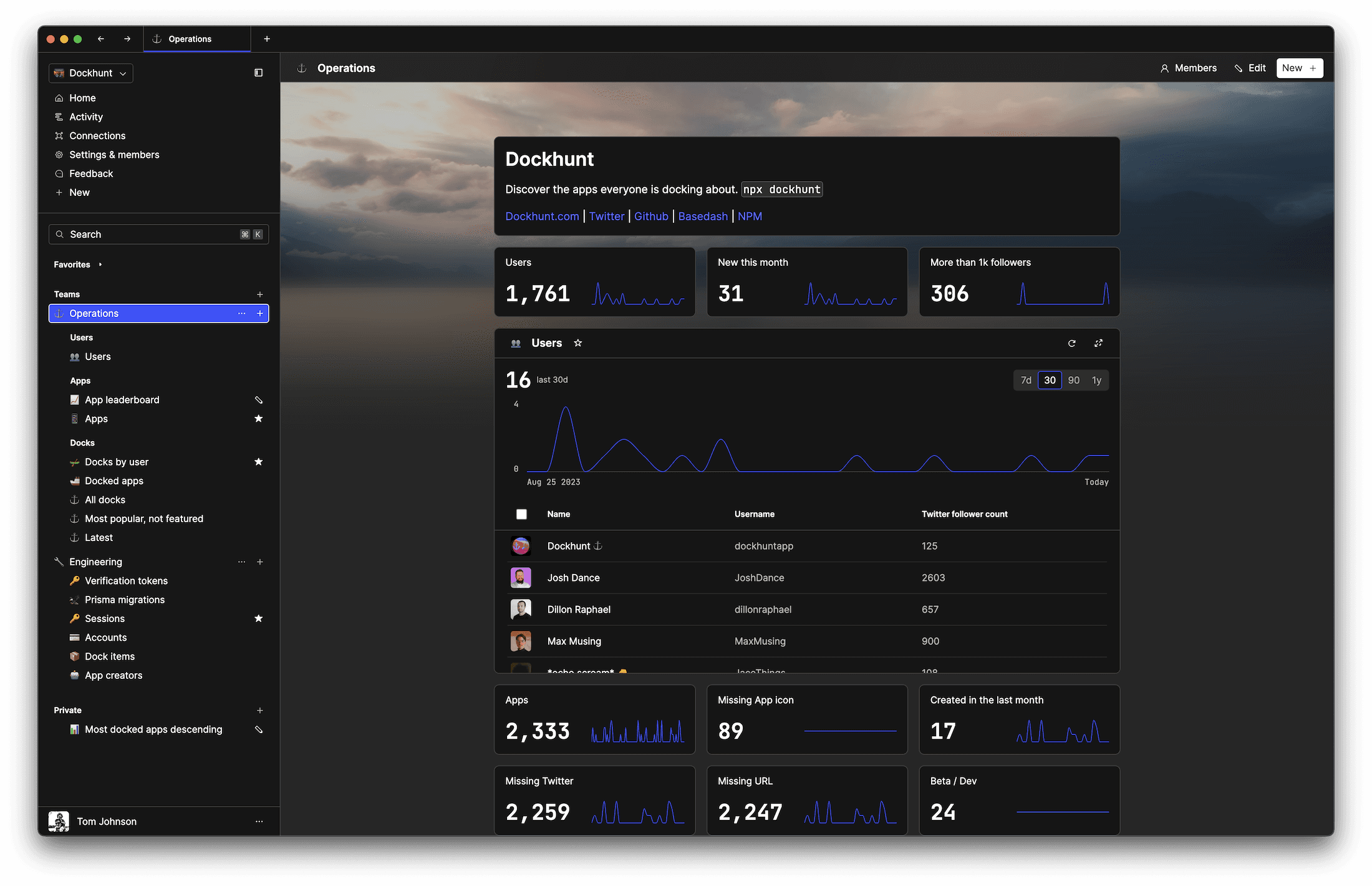SOC II
Type 2 Certified

### Features

Proudly carbon-neutral.SOC II
Type 2 Certified

### Features

Proudly carbon-neutral.SOC II
Type 2 Certified